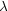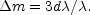Meanwhile it may be pointed out that the individual energies of the quanta reaching us from the nebulae are altered by red-shifts, quite-apart from the question of recession. In view of the fundamental relation previously mentioned,the mere presence of red-shifts, the observed increase in wave-lengths, necessarily implies a reduction in the energies. This energy effect operates regardless of the interpretation of red-shifts. It must be applied as a matter of course to all measured luminosities before distances can be estimated.

The calculation of the energy effect starts from the fact that the fractional red-shift d/is constant throughout a given spectrum. Each wave-length is increased, and, consequently, the energy in each quantum is reduced, by the constant factor 1 + d/. We might naturally conclude that the total luminosity, the sum of all the individual energies, would be reduced by the same factor. The conclusion, in fact, is valid in the particular case of the `bolometric' luminosity, which is the total radiation, integrated over all wave-lengths, as it would be measured in empty space outside the earth's atmosphere. But we are concerned with the photographic luminosity. Hence the effect must be traced through the, atmosphere, the telescope, and the photographic film. The procedure is complicated because the energy effect is selective (varying through the spectrum). The distortions magnify the original factor 1 + d/to a factor that approaches 1 + 3d/. More precisely, the photographic magnitudes, to use the technical measure of apparent faintness, are increased by the increment,This is the correction for the energy effect which is valid regardless of the origin of red-shifts, 2 and which is applied as a matter of course to all measures of apparent luminosity used for the estimation of distances.

2 Since E= constant = h / c, the above statement assumes that the relation between Planck's constant h and the velocity of light c is essentially the same for light from the distant nebulae as in light from laboratory sources. The assumption has been tested by measuring red-shifts both with a grating spectrograph and a prism spectrograph. The fact that the shifts are the same in both cases seems to confirm the constancy of h / c. (Annual Report of the Mount Wilson Observatory, 1935-6.) Back.